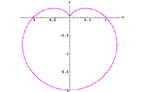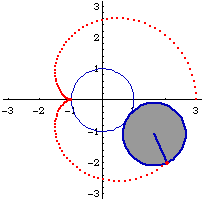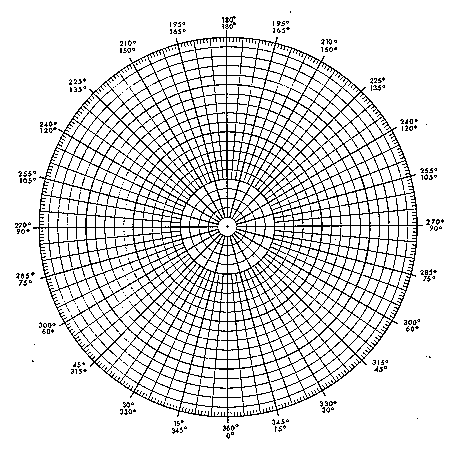Polar Plot - cardioid

Won't you be my Valentine?Introduction

A simple plot in polar coordinates will produce a heart shaped function known as a cardioid.

Material

Mechanical version

Two disk shaped magnets
A piece of paper and a pencil.

Low-tech version

Polar plotting paper (Available in the book "Graph paper from your copier")
A scientific calculator that can compute sine functions

High-tech version

Assembly

Mechanical version

Make a dot on one edge of one of the disk magnets, use ink or white-out, or nail polish.

Low-tech version, none.

High-tech version

Go to

http://mss.math.vanderbilt.edu/cgi-bin/MSSAgent/~pscrooke/MSS/plotpolar.def

or http://www.webmath.com/polar.html

To Do and Notice

Mechanical

Place one of the magnetic disks on a piece of white paper.
Place the second disk next to the first so that it is attracted.
Roll the second disk around the first one. Use the pencil to mark the position of the dot on the second disk as it rolls completely around the first disk once.

Connect the dots to create a cardioid.Low-tech

Have students plot the function r = 1 - sin(T) where r is the radius and T is the angle in degrees with 0<T<360

High-tech

Enter the function 1-sin(t)

and the range tmin= 0, tmax=2*pi, notice that this angle is in radians.

press the plot button.

What's Going On?

The curve you produced is known as a cardioid. A cardioid can be defined as the trace of a point on a circle that rolls around a fixed circle of the same size without slipping.

The magnets are oriented so that they attract each other. The attraction produces enough friction that the magnet can roll without slipping easily.

So What?

The polar plot of the sensitivity of a cardioid microphone, or antenna, is a cardioid.

Etc.

Try variations on this function.

Predict what the graph will look like first.

r = 1 + sin(t)
r = 1 - 1.25 * sin(t)
r = 1
r = 1 + sin(t)
r = 1 + cos(t)
r = 1 - (sin(t))^3
r = 1 - (sin(t))^9
r = 1 - (sin(t))^99

Resources

Sources of information for Cardioids

http://www.best.com/~xah/SpecialPlaneCurves_dir/Cardioid_dir/cardioid.html

http://www-groups.dcs.st-andrews.ac.uk/%7Ehistory/Curves/Cardioid.html

A table of the Sine function is provided below.

Angle t, degrees sin(t)

 0 0 20 0.34 40 0.64 60 0.87 80 0.98 100 0.98 120 0.87 140 0.64 160 0.34 180 0 200 -0.34 220 -0.64 240 -0.87 260 -0.98 280 -0.98 300 -0.87 320 -0.64 340 -0.34 360 0

Polar Graph PaperDraft Activities

 Scientific Explorations with Paul Doherty © 1999 21 Feb 99